# Different Types of Transistor Configuration – Elprocus

We know that transistor consists of three terminals namely emitter, collector and base and these are denoted by E, C and B. But, in the applications of transistors we require four terminals, two terminals for input and remaining two terminals for output. To rectify this problem, we use one terminal for both i/p and o/p actions. Using this concept we design the circuits, which will offer the required characteristics and these configurations are called transistor configurations.

## Types of Transistor Configurations

The three different kinds of transistor configurations are

• Common base transistor configuration
• Common emitter transistor configuration
• Common collector transistor configuration

Now we discuss about the above three transistor configurations with diagrams.

### Common Base Transistor Configuration (CB)

The common base transistor configuration gives a low i/p while giving a high o/p impedance. When the voltage of the CB transistor is high, the gain of the current and overall gain of the power is also low compared to the other transistor configurations. The main feature of the B transistor is that the i/p and o/p of the transistor are in phase. The following diagram shows the configuration of CB transistor. In this circuit, the base terminal is mutual to both i/p & o/p circuits.

The current gain of the CB circuit is calculated in a method related to that of the CE concept and it is denoted with alpha (α). It is the relationship between collector current and emitter current.The current gain is calculated by using the following formula.

Alpha is the relationship of collector current (output current) to emitter current (input current). Alpha is calculated using the formula:

α=(∆Ic )/∆IE

For instance, if the i/p current (IE) in a common base current change from 2mA to 4mA and the o/p current (IC) changes from 2mA to 3.8 mA, the gain of the current will be 0.90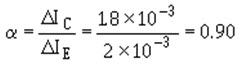The current gain of the CB current is less than 1. When the emitter current flows into the base terminal and doesn’t perform as collector current. This current is always less than the emitter current which causes it. The gain of the common base configuration is always less than 1. The following formula is used to calculate the current gain of the CE (α) when the CB value is given i.e (β).

### Common Collector Transistor Configuration (CC)

The common collector transistor configuration is also known as the emitter follower because the emitter voltage of this transistor follows the base terminal of the transistor. Offering a high i/p impedance & a low o/p impedance are commonly used as a buffer.The voltage gain of this transistor is unity, the current gain is high and the o/p signals are in phase. The following diagram shows the configuration of CC transistor. The collector terminal is mutual to both i/p and o/p circuits.

The current gain of the CC circuit is denoted with (γ) and it is calculated by using the following formula.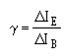This gain is related to CB current gain that is beta (β), and gain of the CC circuit is calculated when the b value is given by the following formula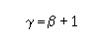When the transistor is connected in any of three basic configurations such as CE, CB and CC then there is a relationship between alpha, beta and gamma. These relations are given below.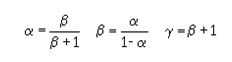For instance, the current gain value of the common base value (α) is 0.90, then the beta value can be calculated as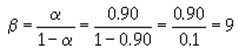Therefore, a variation in base current of this transistor will give a change in collector current that will be nine times as big. If we want to use the same transistor in a CC, we can calculate gamma by the following equation.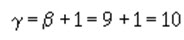### Common Emitter Transistor Configuration (CE)

The common emitter transistor configuration is most widely used configuration. The circuit of CE transistor gives a medium i/p and o/p impedance levels. The gain of the both voltage and current can be defined as a medium, but the o/p is opposite to the i/p that is 1800 change in the phase. This gives a good performance and it is frequently thought of as the most commonly used configurations. The following diagram shows the configuration of CE transistor. In this kind of circuit, the emitter terminal is mutual to both i/p & o/p.

The following table below shows the configurations of common emitter, common base and common collector transistors.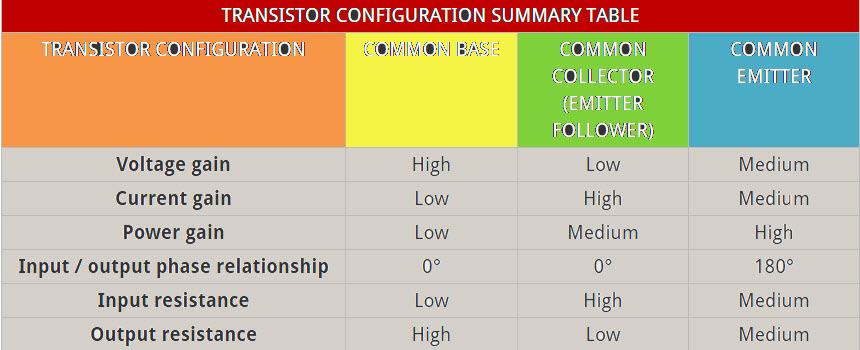The current gain of the common emitter (CE) circuit is denoted with beta (β).It is the relationship between collector current and base current.The following formula is used to calculate the beta (β). Delta is used to specify a small change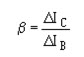For instance, if the i/p current (IB) in a CE change from 50 mA to75 mA and the o/p current (IC) changes from 2.5mA to 3.6mA, the current gain (b) will be 44.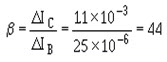From the above current gain, we can conclude that a change in base current generates a change in collector current which is 44 times larger.

This is all about different types of transistor configurations which includes common base, common collector and common emitter. We believe that you have got a better understanding of this concept. Furthermore, any queries regarding this concept or electronics projects, please give your valuable suggestions by commenting in the comment section below.here is a question for you, what is the function of transistor?